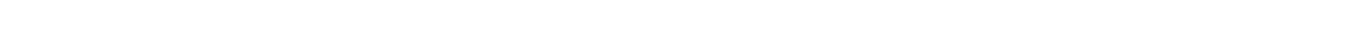# 2.3.4 Feynman Diagrams

### Feynman Diagrams

• Feynman diagrams are a clear way of representing particle interactions in terms of their incoming and outgoing particles and exchange particles
• Although there are many variations of Feynman diagrams, they follow a set of rules:
• The vertical axis represents time
• The horizontal axis represents space
• Gauge bosons are represented by a wavy or dashed lines or a helix
• All other particles are represented by straight lines
• Each line (apart from neutral gauge bosons) must have an arrow with its direction forward in time
• The total charge, baryon number and lepton number must be conserved at each vertex
• Particle lines must not cross overFeynman diagrams follow a set of rules which are needed to interpret them accurately

• Feynman diagrams show nothing about the actual path of the particles, so the angles in the diagram are of no significance
• Note that you might find the following variations of Feynman diagrams:
• The vertical axis representing space and the horizontal axis representing time
• Anti-particles represented as moving backwards in time
• However, it is best to stick to the rules given for this syllabus which have time on the vertical and space on the horizontal axis and all particles moving forward in time

#### Exchange Particles

• Gauge bosons are represented differently in each Feynman diagram depending on the type of interaction
• Charged exchange particles (W+ and W) must have their direction indicated
• Representing exchange particles:
• In the weak interaction, W and Z bosons are represented by a wavy line
• The W+ and W must have an arrow showing their direction (left or right) or must be slanted upwards, meaning that they are forward in time
• Pions are represented by a wavy or dashed line
• Photons are represented by a wavy line
• Gluons are represented by a helixThe gauge bosons are depicted by a wavy or dashed line, or a helix depending on the type of interaction

#### Worked Example

A neutron interacts with a neutrino in the following way:Draw the Feynman diagram that corresponds to the neutron and neutrino interaction represented in the equation.• The vertical axis represents time and the horizontal axis represents space
• All particles are labelled correctly
• All particles are represented by straight lines and arrows pointing forward in time
• W+ boson represented by a wavy line with an arrow pointing towards the neutron-proton vertex

#### Exam Tip

The most common exam mistakes when asked to draw Feynman diagrams are missing out arrows indicating the direction of charged gauge bosons or particles. Although you are not required to sketch and label the space and time axes, all particles must be labelled accurately.

### Quark Transformation in β decay

• β decay occurs because of the weak interaction between quarks

#### Quark Composition: β– decay

• β decay is when a neutron turns into a proton emitting an electron and anti-electron neutrino
• More specifically, a neutron turns into a proton because a down quark turns into an up quark
• The W boson ‘carries away’ the negative charge of the down quark which provides the negative charge for the electron and anti-neutrinoIn beta minus decay, the weak interaction turns a down quark into an up quark

#### Quark Composition: β+ decay

• β+ decay is when a proton turns into a neutron emitting an positron and an electron neutrino
• More specifically, a proton turns into a neutron because an up quark turns into a down quarkBeta minus decay is when an up quark turns into a down quark

• The W+ boson ‘carries away’ the positive charge of the up quark which provides the positive charge for the positron and neutrinoIn beta plus decay, the weak interaction turns an up quark into a down quark### Author: Ashika

Ashika graduated with a first-class Physics degree from Manchester University and, having worked as a software engineer, focused on Physics education, creating engaging content to help students across all levels. Now an experienced GCSE and A Level Physics and Maths tutor, Ashika helps to grow and improve our Physics resources.
CloseClose